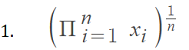# Arithmetic Mean: Definition How to Find it

Statistics Definitions > Arithmetic Mean

Contents:

## What is the Arithmetic Mean?

The arithmetic mean is another name for the mean or the average. When someone talks about the mean of a data set, they are usually talking about the arithmetic mean (most people just drop the word “arithmetic”). It’s called a different name to set it apart from other means found in math, including the geometric mean.

The mean is influenced by outliers , so it isn’t always a good indicator of where the middle of a data set is. For data sets that have either a lot of low values or a lot of high values, the median is often a better way to describe the “middle.”

## How to find the Arithmetic Mean

Finding the arithmetic mean takes two steps: add all the numbers up and then divide by the number of items in your set. The arithmetic mean is found in the exact same way as a sample mean (“sample” here just means a number of items in your data set). Watch the video, or read the steps below:

Example problem: Find the arithmetic mean for average driving speed for one car over a 6 hour journey: 54 mph, 57 mph, 58 mph, 66 mph, 69 mph, 71 mph

Step 1: Add all the numbers up: 54 + 57 + 58 + 66 + 69 + 71 = 375.

Step 2: Divide by the number of items in the set. For this set there are 6 numbers, so:
375 / 6 = 62.5.

Solution: The average driving speed is 62.5 mph.

For another example with steps, see the next article:
How to find the mean

## Population vs. Sample Mean

If your data is a population, then the mean is called a population mean, represented by the letter μ. If the list is a sample, it’s called a sample mean x̄.

## Showing the Arithmetic Mean is Greater than the Geometric Mean

Jensen’s inequality, usually taught in a calculus based statistics course, can be used to show that the arithmetic mean of n positive scalars x1,x2,…xn, is greater than or equal to their geometric mean, which is equal toWhere Π is product notation.

This statement can be shown by considering a convex function f(x) = -log x. Let X be a discrete random variable with values x1,x2,…xn, and probabilities 1/n :By Jensen’s inequality:
E (-log X) ≥ –log E(X).

Check out our YouTube channel for hundreds of statistics and calculus videos.

## References

 Khuri, A. Advanced Calculus with Applications in Statistics. Second Edition. Wiley.

CITE THIS AS:
Stephanie Glen. "Arithmetic Mean: Definition How to Find it" From StatisticsHowTo.com: Elementary Statistics for the rest of us! https://www.statisticshowto.com/arithmetic-mean/